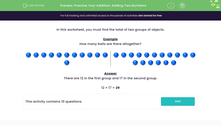Comprehensive & curriculum aligned

In this worksheet, students find the total of two groups of objects by counting them.Key stage:  KS 1

Curriculum topic:   Number: Addition and Subtraction

Curriculum subtopic:   Solve Add/Subtract Problems to 100

Difficulty level:Worksheet Overview

In this worksheet, you must find the total of two groups of objects.

Example

How many balls are there altogether?There are 12 in the first group and 17 in the second group.

12 + 17 = 29

What is EdPlace?

We're your National Curriculum aligned online education content provider helping each child succeed in English, maths and science from year 1 to GCSE. With an EdPlace account you’ll be able to track and measure progress, helping each child achieve their best. We build confidence and attainment by personalising each child’s learning at a level that suits them.

Get started